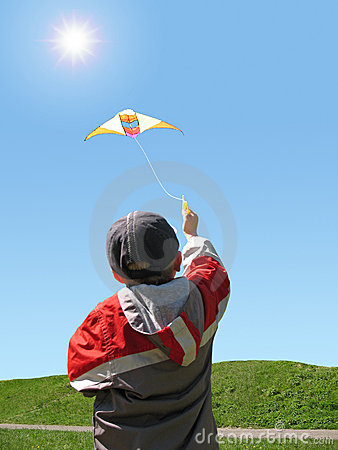# Always had a desire to fly kite with sosI am flying a kite. The kite is moving horizontally at $151.5m$ above the ground. If the speed of the kite is $10m/s$. How fast (in m/s) is the string being let out,when the kite is $250m$ from me ? (assuming my height to be $1.5m$) ?

×